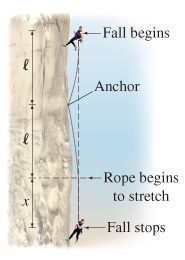# Problem: Stretchable ropes are used to safely arrest the fall of rock climbers. Suppose one end of a rope with unstretched length l is anchored to a cliff and a climber of mass m is attached to the other end. When the climber is a height l above the anchor point, he slips and falls under the influence of gravity for a distance 2l, after which the rope becomes taut and stretches a distance exttip{x}{x} as it stops the climber (see the figure). Assume a stretchy rope behaves as a spring with spring constant exttip{k}{k}.Applying the work-energy principle, find x.Assuming exttip{m}{m_0} = 86 kg , exttip{l}{l_0} = 8.6 m and exttip{k}{k_0} = 870 N/m , determine x/l (the fractional stretch of the rope) at the moment the climbers fall has been stopped.Assuming exttip{m}{m_0} = 86 kg , exttip{l}{l_0} = 8.6 m and exttip{k}{k_0} = 870 N/m , determine kx/mg (the force that the rope exerts on the climber compared to his own weight) at the moment the climbers fall has been stopped.

###### FREE Expert Solution
80% (420 ratings)
###### Problem Details

Stretchable ropes are used to safely arrest the fall of rock climbers. Suppose one end of a rope with unstretched length l is anchored to a cliff and a climber of mass m is attached to the other end. When the climber is a height l above the anchor point, he slips and falls under the influence of gravity for a distance 2l, after which the rope becomes taut and stretches a distance as it stops the climber (see the figure). Assume a stretchy rope behaves as a spring with spring constant .

Applying the work-energy principle, find x.

Assuming = 86 kg , = 8.6 m and = 870 N/m , determine x/l (the fractional stretch of the rope) at the moment the climbers fall has been stopped.

Assuming = 86 kg , = 8.6 m and = 870 N/m , determine kx/mg (the force that the rope exerts on the climber compared to his own weight) at the moment the climbers fall has been stopped.

Frequently Asked Questions

What scientific concept do you need to know in order to solve this problem?

Our tutors have indicated that to solve this problem you will need to apply the Work By Variable Forces (Springs) concept. You can view video lessons to learn Work By Variable Forces (Springs). Or if you need more Work By Variable Forces (Springs) practice, you can also practice Work By Variable Forces (Springs) practice problems.